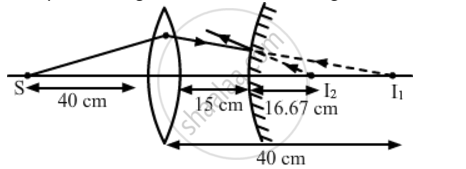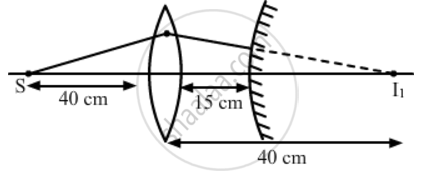# A Convex Lens of Focal Length 20 cm is Placed Coaxially with a Convex Mirror of Radius of Curvature 20 cm. the Two Are Kept 15 Cm Apart. a Point Object is Placed 40 Cm in Front of the Convex Lens. Find the Position of the Image Formed by this Combination. Draw the Ray Diagram Showing the Image Formation. - Physics

A convex lens of focal length 20 cm is placed coaxially with a convex mirror of radius of curvature 20 cm. The two are kept 15 cm apart. A point object is placed 40 cm in front of the convex lens. Find the position of the image formed by this combination. Draw the ray diagram showing the image formation.

#### Solution

Let us first locate the image of the point object S formed by the convex lens.

Here:
u = -40 cm

And, f = 20 cm

From the lens formula, we have:

1/v-1/u=1/f

=>1/v=1/f+1/u

=>1/v=1/20+1/(-40)

=>1/v=(2-1)/40=1/40

⇒ 40 cm

The positive sign shows that the image is formed to the right of the lens as shown in the following figure.The image I1 is formed behind the mirror and hence acts as a virtual source for the mirror. The convex mirror forms the image I2, whose distance from the mirror can be calculated as:

1/v+1/u=1/f

Here:

u = 25 cm

And  f= R/2=10 cm

=>1/v=1/f-1/u

=>1/v=1/10-1/25

=>1/v=(5-2)/50=3/50

⇒ 16.67 cm

Hence, the final image is formed at a distance of 16.67 cm from the convex mirror as shown in the following figure.'Concept: Concave Mirror
Is there an error in this question or solution?
2013-2014 (March) All India Set 3

Share